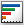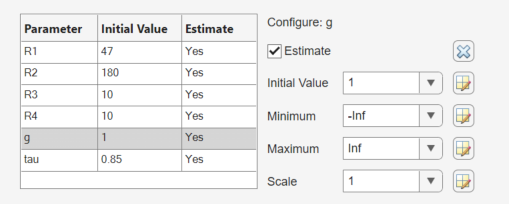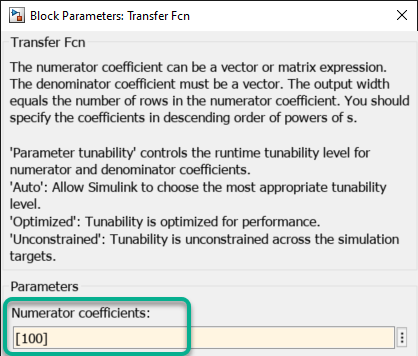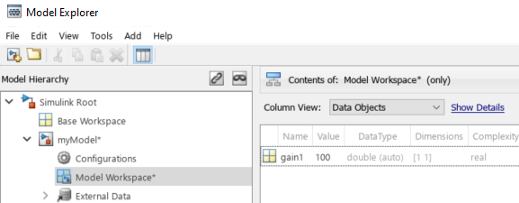## Specify Parameters for Estimation

To perform parameter estimation, in addition to specifying experiment data, you must also identify the model parameters that the optimizer can modify.

Design variables in Simulink® Design Optimization™ software can take scalar, vector, or matrix values, or be specified as an expression that evaluates to such a value. Simulink Design Optimization software lets you estimate scalar, vector, and matrix parameters. Parameters can vary continuously, or take values restricted to a discrete, finite set. When you specify parameters at the command line or in the Parameter Estimator app, you designate each parameter as either continuous or discrete valued. For continuous parameters, you can specify parameter bounds for the optimization. For discrete parameters, you specify the set of allowed values, which can be numeric or string values.

### Choose Parameters to Estimate

It can be useful to take an iterative approach to estimating model parameters. For example, if you have a large number of parameters to estimate, start by estimating those that most influence the output. After you estimate a subset of parameters and validate the estimated parameters, you can select the remaining parameters for estimation.

To identify the parameters that most influence the estimation and focus your optimization on those parameters, you can use sensitivity analysis. In the Parameter Estimator, you can access the Sensitivity Analyzer app by clickingSensitivity Analysis. In the Sensitivity Analyzer app, you can explore the response optimization design space, identify the parameters that most influence the optimization problem, and compute initial values. Then, return to the Parameter Estimation app and use the most sensitive variables for your optimization. For more information, see Identify Key Parameters for Estimation (GUI) and Use Sensitivity Analysis to Configure Estimation and Optimization.

### Specify Parameters for Estimation

You can specify the parameters for estimation experiments using the Estimated Parameters editor. In the Parameter Estimator, on the Parameter Estimation tab, clickSelect Parameters. This action opens the Edit: Estimated Parameters dialog box.To select parameters that apply to all experiments, click in the Parameters Tuned for all Experiments panel. Then, select and configure parameters as described in Select Parameters Using Select Model Variables Dialog Box.

If you have assigned variables to a specific experiment as described in Specify Estimation Data, those variables appear in the Parameters and Initial States Tuned per Experiment. Use this table to configure parameters specifically for this experiment. Use the table as described in Select Parameters Using Select Model Variables Dialog Box.

### Select Parameters Using Select Model Variables Dialog Box

In the Select Model Variables dialog box, the table lists the variables that the model uses to set block parameter values. These variables can reside in the model workspace, the base workspace, or a data dictionary.

Select variables by checking the box next to each variable. In Parameter Estimator, you can specify whether a parameter is continuous or discrete.

• Select Continuous to specify a continuously variable parameter.

• Select Discrete to specify a parameter whose values during optimization are limited to a discrete, finite set. (since R2023a)

If your model contains many variables, filter the list by typing in the Filter by variable name field. The Used By column lists all blocks in the model that use the variable. When a variable is used in more than one block, all blocks are listed. To highlight blocks in the model that use the variable, click the block name. When you have completed your selections, click OK.

Note

You can only optimize variables that are in use by the Simulink model. If the model parameter you want to tune does not appear in the list, see Add Model Parameters as Variables for Estimation.

The continuous variables that you select must have a numeric value that uses the data type `double`. Discrete variables can take numeric or string values. If the value of a variable does not meet these conditions, use these techniques:

• To select a single element or a subset of a matrix or array variable, click Specify expression indexing if necessary.Enter an expression such as `myArray(2)`, which selects the second element of an array variable `myArray`.

After you type the expression, press the Enter key to add the variable to the list of model variables.

• To use a variable of a numeric data type other than `double`, convert the variable to a `Simulink.Parameter` object, which separates a parameter value from its data type. Set the `Value` property to a default `double` number, and use the `DataType` property to control the data type.

• To use the value of a `Simulink.Parameter` object, specify the `Value` property. Enter the expression `myParamObj.Value`.

• To use a field of a structure, enter `myStruct.PID.P1`. If you store the structure in a `Simulink.Parameter` object, enter `myStruct.Value.PID.P1`.

• To use one cell of a cell array, enter `myCells{3}`.

You cannot use mathematical expressions such as a + b. Sometimes, models have parameters that are not explicitly defined in the model itself. For example, a gain `k` could be defined in the MATLAB® workspace as `k = a + b`, where `a` and `b` are not defined in the model but `k` is used. To add these independent parameters, see Add Model Parameters as Variables for Estimation.

### Specify Initial Values, Bounds, and Allowed Values

After you select parameters, you can specify initial values, variable bounds (for continuous parameters) and sets of allowed values (for discrete parameters) in the Edit: Estimated Parameters dialog box or the Edit Experiment dialog box. In the table, select the parameter you want to edit, and use the text fields or variable editor to change the values. Clear the Estimate checkbox to exclude the parameter from the current estimation.

#### Continuous Parameters

For continuous parameters, specify the initial value, range, and scale factor.• Initial Value — Initial value for estimation. By default, the initial value is the current parameter value in the model. If you change the initial value in the table, click to update the values in the model.

• Minimum and Maximum — Lower and upper bounds on the value the parameter can take during estimation, respectively. The defaults are `-Inf` and `Inf`.

Often, there are computational advantages in specifying finite bounds. Furthermore, it can be important to specify finite bounds to get meaningful results. For example, if a parameter specifies a quantity that must be positive (such as the mass of a physical object), specify `0` as the absolute lower bound even if better information is unavailable.

• Scale — Scaling factor. During optimization, the design variables are scaled, or normalized, by dividing their current value by a scale value. The default value is the nearest power of 2 greater than the current value of the parameter. Specifying a scaling factor can be useful when your parameters are separated by several orders of magnitude.

#### Discrete Parameters

Since R2023a

For discrete parameters, specify the initial value and the finite set of values that the discrete parameter can take during estimation.• Value — Initial value for estimation. By default, the initial value is the current parameter value in the model. The initial value must be an element of the value set. If you change the initial value in the table, click to update the values in the model.

• Value Set — Vector of values that the parameter can take during estimation. The default value is the current value of the parameter. Edit this field to specify allowed values. Discrete parameters are not limited to integer or evenly spaced values, and can be string values. For example, `[1.0,1.5,3.0,5.5]`, `1:10`, or `["off","low","high"]`. If you specify a value set that does not include the initial value, then the Value field updates to the first element of the value set.

Note

Estimating discrete parameters requires using the surrogate optimization method. To specify this method in the app, select Options > Optimization. Then, in the Response Optimization Options dialog box, in the Optimization tab, in the Method menu, select `Surrogate optimization`. When you use this method, all continuous parameters must have finite bounds.

### Add Model Parameters as Variables for Estimation

The software can only estimate variables that are in use by the model. Create variables for estimation in the MATLAB or model workspace, and specify your Simulink model or block parameters using these variables.

In this figure, the Numerator coefficients parameter of a Transfer Fcn block is specified as a numerical value.To estimate the Numerator coefficients parameter, specify it as variable `gain1`:

1. Create the variable `gain1` in one of the following ways:

• Add the variables to the model workspace, and specify initial values.• Write initialization code in the PreloadFcn callback of the model. For more information, see Model Callbacks.

`gain1 = 100`

2. Specify the block parameter as variable `gain1` in the Transfer Fcn block dialog box.You can now select `gain1` for estimation. See Specify Parameters for Estimation.

#### Specify Independent Parameters for Estimation

You can also specify independent parameters that do not appear explicitly in the model as variables for estimation. This workflow is not available with Simulink fast restart.

Suppose that a model parameter `Kint` is related to independent parameters `x` and `y` such that ```Kint = x+y```. To estimate `x` and `y` instead of `Kint`:

• Create the independent variables `x` and `y` by adding them to the model workspace and specifying initial values.

• The software only allows tuning of variables that are used by model blocks. To ensure that the software detects `x` and `y` for tuning, add a Constant block to your model, and specify the Constant value of the block as `[x y]`. Connect the block to a Display block.• Write code in the InitFcn callback of the model that defines the relationship between `Kint`, `x`, and `y`. You must first use the `get_param` function to get the variables `x` and `y` from the model workspace before you can use them to define `Kint`.

```wks = get_param(gcs,'ModelWorkspace') x = evalin(wks,'x') y = evalin(wks,'y') Kint = x+y; ```

You can now select `x` and `y` for estimation. Do not estimate the independent and dependent parameters simultaneously. Doing so can lead to incorrect results. For example, do not estimate `Kint`, `x` and `y` together.